## NUMBERS AND OPERATIONSThe addition is the mathematical composition operation that consists of combining, joining or adding two or more numbers to obtain a final or total quantity.

In the additive expression, four fundamental properties are always satisfied:

1) Commutative property:

A modification in the order of its elements (addends) will not modify the result; for example: 3 + 2 = 2 + 3

2) Associative property:

When three or more numbers are added, the order of the addends does not influence the result; for example: 4 + (2 + 1) = (4 + 2) + 1

3) Property of the neutral element (or identity element):

Any number added to the number zero (0) will result in the same number; for example: 5 + 0 = 5

4) Distributive property (combining addition and multiplication):

The result of the sum of two numbers (A and B) multiplied by a third number (C) will be equivalent to the sum of their products (A x C + B x C); for example: 2 x (4 + 3) = 2 x 4 + 2 x 3

What is addition for children and how do we teach it?

To correctly understand what a sum is, we provide you with a visual example where you have to count the number of elements in each set (set A and B ) and check the result obtained when we group them (A U B set):

SPANISHENGLISHTherefore, we can say that "add" consists of joining or uniting the elements of various sets to form a single set.

What do the symbols "+" and "=" mean in a sum?

- The symbol "+" is read "plus", "and" or "added to" and is always inserted between the elements that we have to add.

Example: The addition sentence 4 + 2 is read "four plus two"

- The symbol "=" is read "equals", "equal", "is" or "are" and represents the result that we obtain after joining, joining or grouping the elements of two sets in a single set.

Example: The solved sum 4 + 2 = 6 is read "four plus two equals six"

SPANISHENGLISH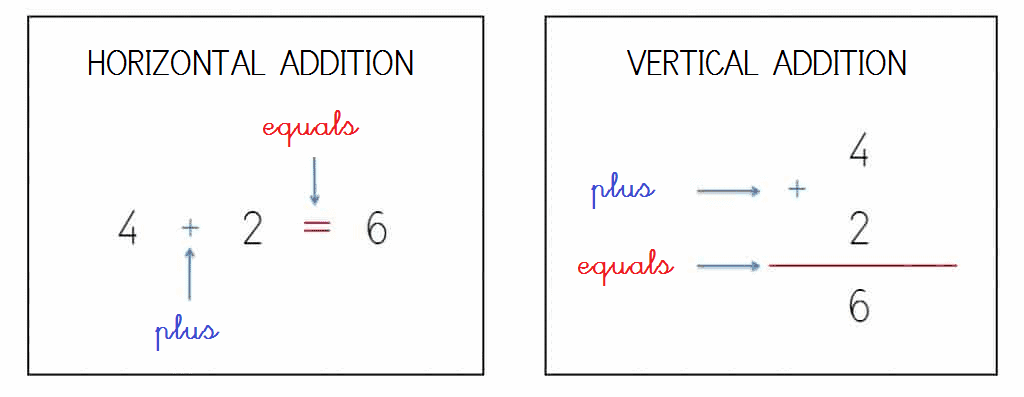#### Know the elements of addition and their position with natural numbers up to 49

What are the "addends" and the "result" in a sum?

- Addends: They represent each of the figures or numbers that we are going to add, that is, they correspond to the sets that we will join, combine and / or group.

- Result or Sum: Symbolizes the figure or number resulting from the sum. It corresponds to the unique set formed by joining, combining and / or grouping the quantities of the addends.

SPANISHENGLISH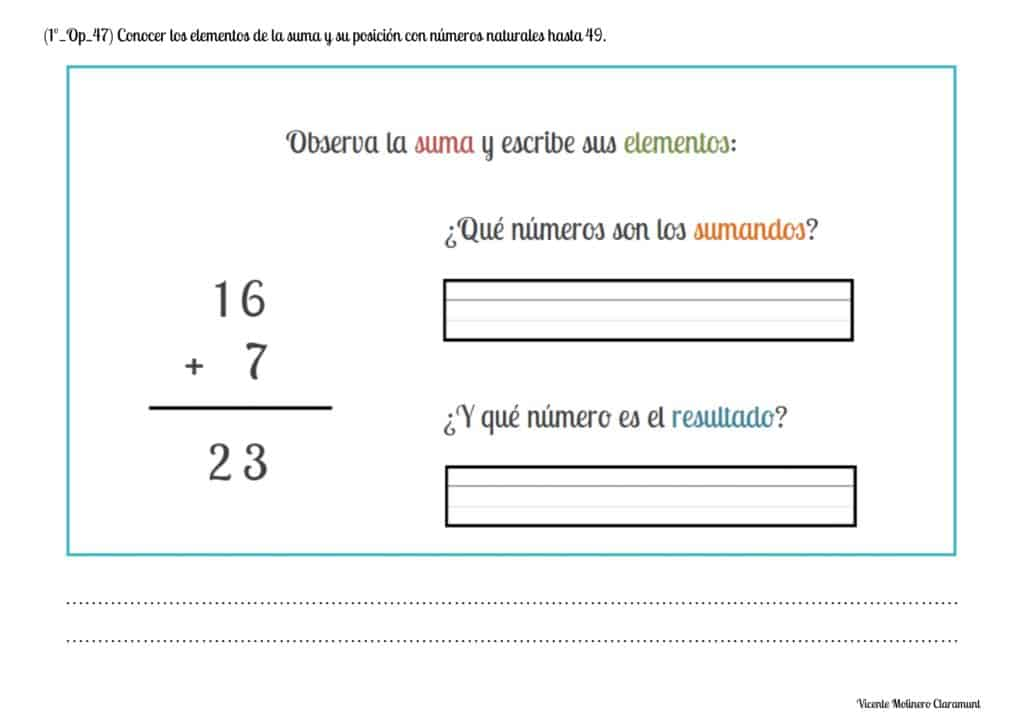#### Properly place the terms of the addition in natural numbers up to 49

Next you will learn to construct vertical sums by placing the symbols "+" and "=" and each of their terms (addends and result) in the corresponding place, practicing with the different printable educational worksheets that we have developed for whole numbers from 0 to 50.#### Solve sums of two addends vertically without regrouping with natural numbers up to 49

How to add two-digit numbers no carrying?

We present you some printable PDF activities accompanied by their explanation and an educational game so that you can easily learn how to add numbers from 0 to 50 vertically and without regrouping.

SPANISHENGLISH#### Solve sums of two addends vertically with regrouping with natural numbers up to 49

How to add two-digit numbers carrying?

Practice the 2-digits addition with regrouping with the whole numbers from 0 to 50 from our printable worksheets and become a mathematical expert.

If you need a little preparation, watch our video lessons where we provide you with some solved examples using the column method.

SPANISHENGLISH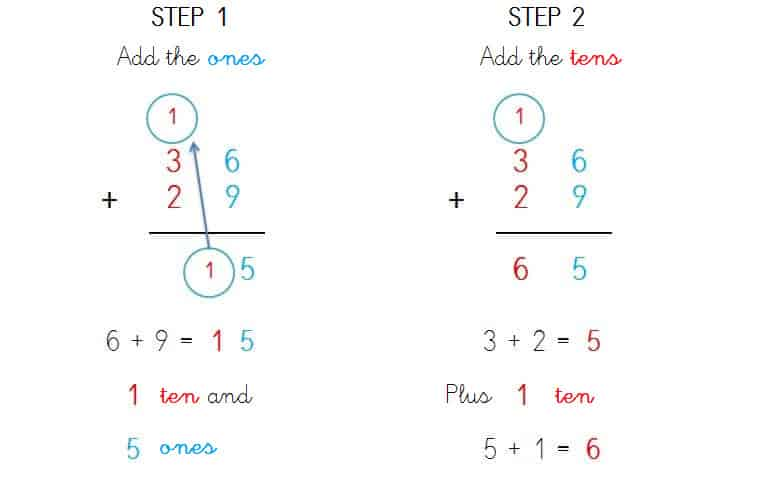#### Solve sums of two addends horizontally without regrouping with natural numbers up to 49

Learn to solve horizontal sums without regrouping with whole numbers from 0 to 49 with our didactic exercises starting from the largest number and then jumping forward on a number line (either real or imaginary).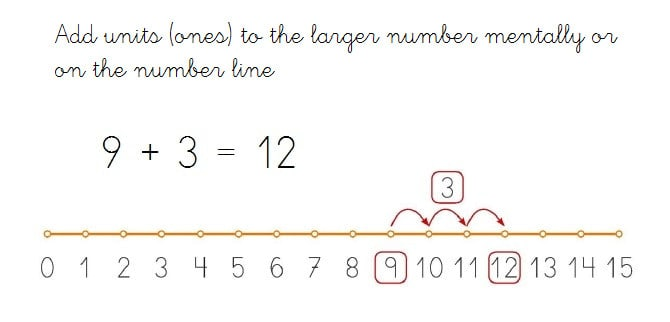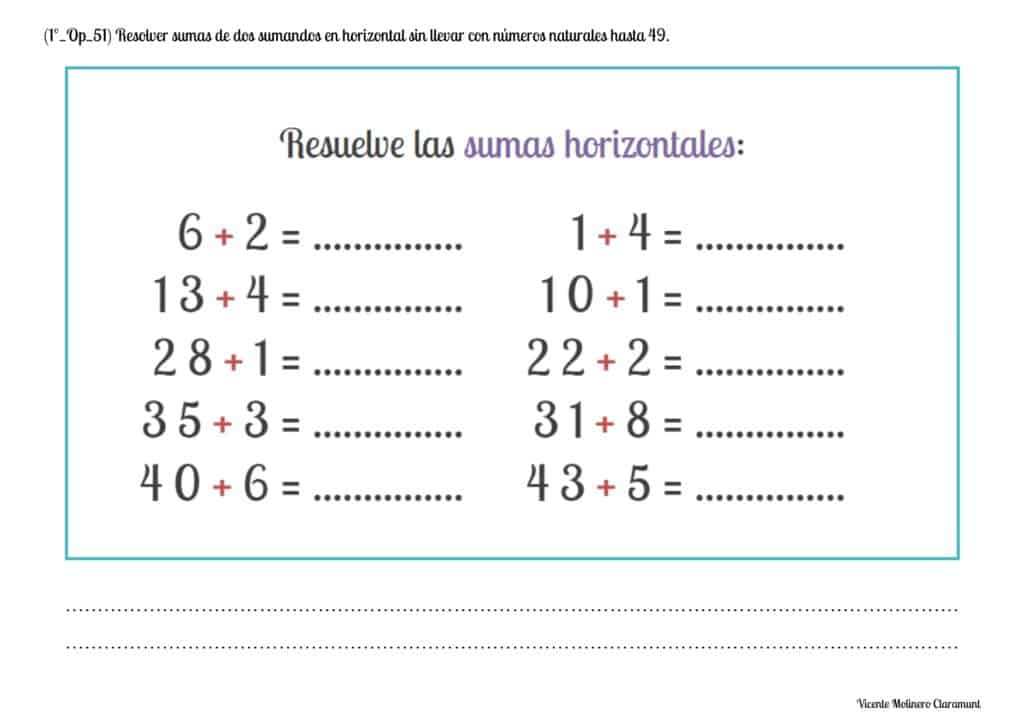#### Solve sums of two addends horizontally with regrouping with natural numbers up to 49

To solve horizontal sums carrying with numbers up to 50 , we will add the ones (for example, 7 + 5 = 12) and, later, we will add the numbers of the tens (2 + 3) and we will include a third adding with the "figure that we carry" of the ones (2 + 3 + 1 = 6).#### Solve sums of three addends vertically without regrouping with whole numbers up to 49

How to add 3 or more numbers without carrying?

We explain step by step how to perform the calculations and how to find the result easily in the additions with three addends no regrouping.

Furthermore, we propose an interactive game so that you can quickly understand the algorithm of addition with three addends.

SPANISHENGLISH1) We will align the numbers according to the place value of their figures, that is, we will align the ones (1s) with the ones and the tens (10s) with the tens.

2) For the ones (1s), we will select a pair of numbers and add them together (for example, 1 + 3 = 4) and, to the result, we will add the third addend (4 + 4 = 8).

3) We will repeat the same process for the tens (10s), we will select a pair of numbers and we will add them together (2 + 3 = 5) and, to the result, you We will add the third addend (5 + 1 = 6).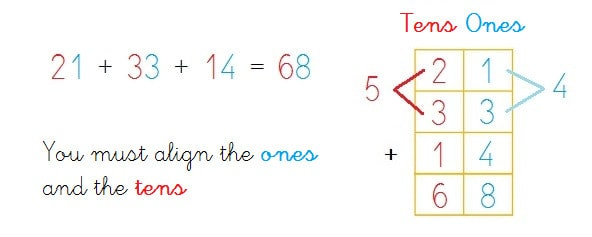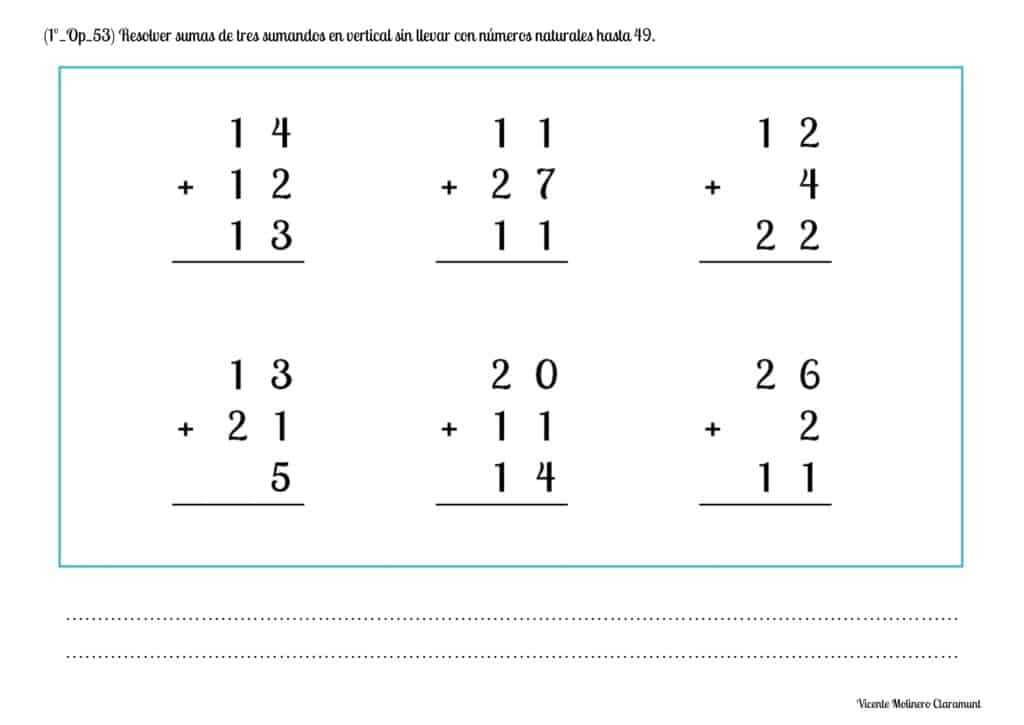#### Solve sums of three addends vertically with regrouping with whole numbers up to 49

How to add 3 or more numbers with carrying?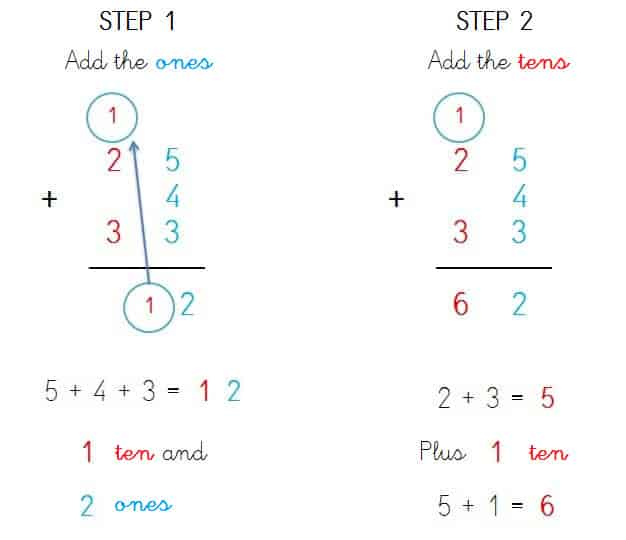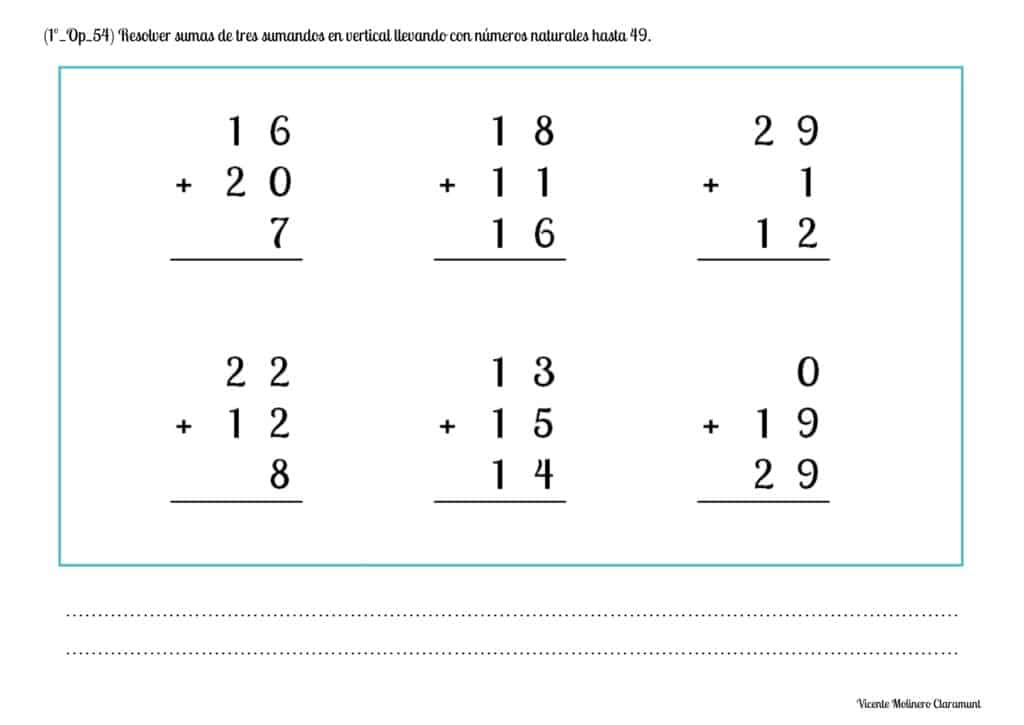#### Recognize that two addends can be added in any order up to 49

Do you know what the Commutative Property of Addition is?

The Commutative Property of Addition is that universal mathematical law that states that "even if we change the order in which the addends are added, the result is always the same".

SPANISHENGLISH#### Recognize that three addends can be added in any order up to 49

Do you know what the Associative Property of the Addition is?

The Associative Property of addition is that universal mathematical law that tells us that "if we add three or more numbers, the result will always be the same regardless of the order in which we group the addends".

SPANISHENGLISH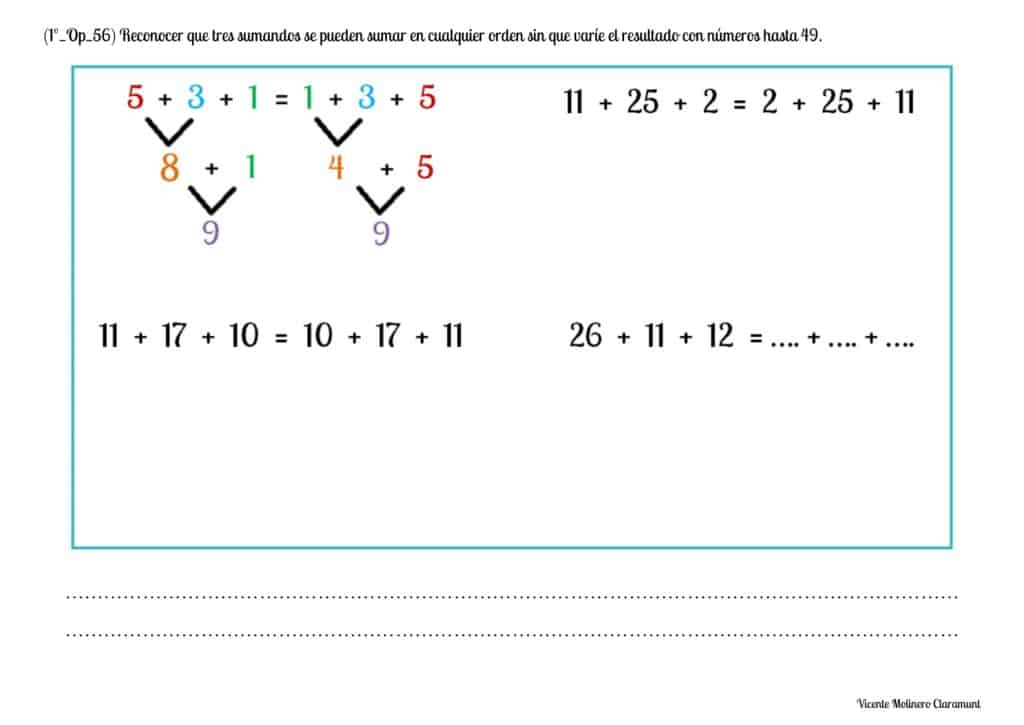#### Mentally solve simple addition facts with natural numbers up to 49

Show your logical-mathematical skills by solving our exercises with hidden or missing whole numbers and using mental calculation (also known as "mental math").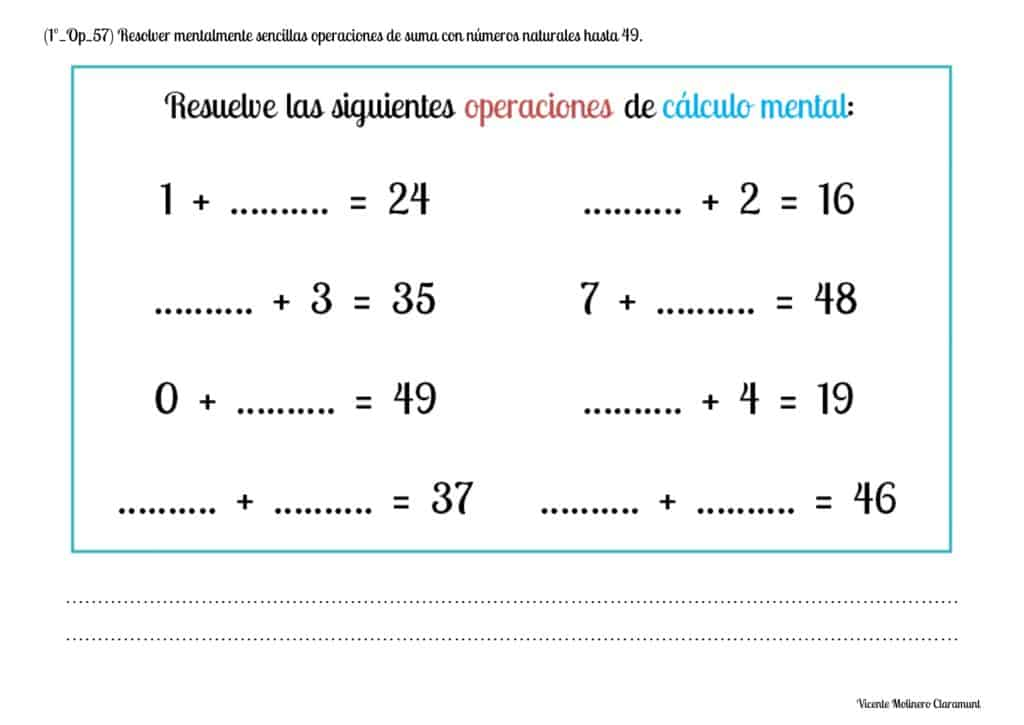#### Solve addition word problems without regrouping with natural numbers up to 49

We have made some exercises so that you can practice solving addition word problems no carrying with the numbers from 0 to 50.

To make it easier for you, we provide you a step-by-step explanation through a video tutorial with 2 digit word problems without regrouping.

SPANISHENGLISH#### Solve addition word problems with regrouping with whole numbers up to 49

Show that you are a math genius by solving each of our arithmetic problems using the basic operation of addition with carryover from 0 to 50.

SPANISHENGLISH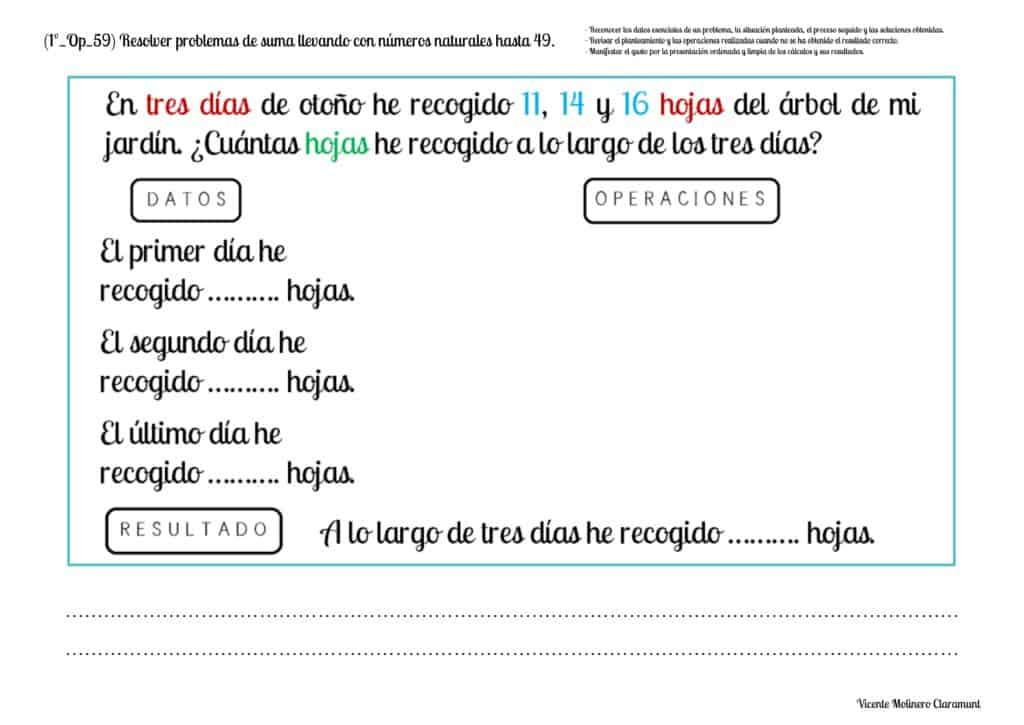Select more exercises, worksheets and activities of Mathematics for each of the 4 learning blocks of the Educational Curriculum ("Numbers and Operations", "Quantities and Measurements", "Geometric and Spatial Reasoning" and "Data Analysis and Probability") aimed at improving the logical-mathematical competencies and skills that are developed throughout "Grade 1" or "Year 2":